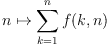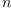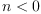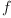login  home  contents  what's new  discussion  bug reports help  links  subscribe  changes  refresh  edit

 Submitted by : (unknown) at: 2007-11-17T22:03:45-08:00 (15 years ago) Name : Axiom Version : default friCAS-20090114 Axiom-20050901 OpenAxiom-20091012 OpenAxiom-20110220 OpenAxiom-Release-141 Category : Axiom Aldor Interface Axiom Compiler Axiom Library Axiom Interpreter Axiom Documentation Axiom User Interface building Axiom from source lisp system MathAction Doyen CD Reduce Axiom on Windows Axiom on Linux Severity : critical serious normal minor wishlist Status : open closed rejected not reproducible fix proposed fixed somewhere duplicate need more info Optional subject :   Optional comment :

## differentiating sums with respect to a bound is wrong

The functionis well defined only for integral values ofgreater than or equal to zero. There is not even consensus how to define this function for. Thus, it is not differentiable. The fix I propose is thus

dvdpatch2.patch

This fix obsoletes the discussion from here on...

Mathematically axiom produces correct output now, however I'm not sure whether my patch is best possible.

Maybe there should be a function D in OutputForm? that displays unevaluated differentiation. Also, I find it ugly to use the raw %diff operator in COMBF. Furthermore, is it necessary to substitute a dummy variable for the variable of differentiation?

Update of Savannah patch # 3148 (project axiom):

Mon 06/21/2004 at 11:15, comment # 1:

  Also, it does not fix bug #9218
Martin Rubey <kratt6>


Tue 12/28/2004 at 14:35, comment # 2:

  What is the status of this patch?
Bill Page <billpage1>
Project AdministratorIn charge of this item.


Continuation of issue #179

dvdpatch.patch

## Compile and Test

SPAD files for the functional world should be compiled in the following order:

    op  kl  function  funcpkgs  manip  algfunc
elemntry  constant  funceval  COMBFUNC  fe


\begin{axiom}
)abbrev category COMBOPC CombinatorialOpsCategory
++ Category for summations and products
++ Author: Manuel Bronstein
++ Date Created: ???
++ Date Last Updated: 22 February 1993 (JHD/BMT)
++ Description:
++   CombinatorialOpsCategory is the category obtaining by adjoining
++   summations and products to the usual combinatorial operations;
CombinatorialOpsCategory(): Category ==
CombinatorialFunctionCategory with
factorials : $->$
++ factorials(f) rewrites the permutations and binomials in f
++ in terms of factorials;
factorials : ($, Symbol) ->$
++ factorials(f, x) rewrites the permutations and binomials in f
++ involving x in terms of factorials;
summation  : ($, Symbol) ->$
++ summation(f(n), n) returns the formal sum S(n) which verifies
++ S(n+1) - S(n) = f(n);
summation  : ($, SegmentBinding$)  -> $++ summation(f(n), n = a..b) returns f(a) + ... + f(b) as a ++ formal sum; product : ($, Symbol)            -> $++ product(f(n), n) returns the formal product P(n) which verifies ++ P(n+1)/P(n) = f(n); product : ($, SegmentBinding  $) ->$
++ product(f(n), n = a..b) returns f(a)  ...  f(b) as a
++ formal product;
)abbrev package COMBF CombinatorialFunction
++ Provides the usual combinatorial functions
++ Author: Manuel Bronstein
++ Date Created: 2 Aug 1988
++ Date Last Updated: 14 December 1994
++ Description:
++   Provides combinatorial functions over an integral domain.
++ Keywords: combinatorial, function, factorial.
++ Examples:  )r COMBF INPUT
CombinatorialFunction(R, F): Exports == Implementation where
R: Join(OrderedSet, IntegralDomain)
F: FunctionSpace R  OP  ==> BasicOperator
K   ==> Kernel F
SE  ==> Symbol
O   ==> OutputForm
SMP ==> SparseMultivariatePolynomial(R, K)
Z   ==> Integer
POWER       ==> %power
OPEXP       ==> exp
SPECIALDIFF ==> "%specialDiff"
SPECIALDISP ==> "%specialDisp"
Exports ==> with
belong?    : OP -> Boolean
++ belong?(op) is true if op is a combinatorial operator;
operator   : OP -> OP
++ operator(op) returns a copy of op with the domain-dependent
++ properties appropriate for F;
++ error if op is not a combinatorial operator;
""       : (F, F) -> F
++ a  b is the formal exponential a*b;
binomial   : (F, F) -> F
++ binomial(n, r) returns the number of subsets of r objects
++ taken among n objects, i.e. n!/(r!  (n-r)!);
permutation: (F, F) -> F
++ permutation(n, r) returns the number of permutations of
++ n objects taken r at a time, i.e. n!/(n-r)!;
factorial  : F -> F
++ factorial(n) returns the factorial of n, i.e. n!;
factorials : F -> F
++ factorials(f) rewrites the permutations and binomials in f
++ in terms of factorials;
factorials : (F, SE) -> F
++ factorials(f, x) rewrites the permutations and binomials in f
++ involving x in terms of factorials;
summation  : (F, SE) -> F
++ summation(f(n), n) returns the formal sum S(n) which verifies
++ S(n+1) - S(n) = f(n);
summation  : (F, SegmentBinding F)  -> F
++ summation(f(n), n = a..b) returns f(a) + ... + f(b) as a
++ formal sum;
product    : (F, SE) -> F
++ product(f(n), n) returns the formal product P(n) which verifies
++ P(n+1)/P(n) = f(n);
product    : (F, SegmentBinding  F) -> F
++ product(f(n), n = a..b) returns f(a)  ...  f(b) as a
++ formal product;
iifact     : F -> F
++ iifact(x) should be local but conditional;
iibinom    : List F -> F
++ iibinom(l) should be local but conditional;
iiperm     : List F -> F
++ iiperm(l) should be local but conditional;
iipow      : List F -> F
++ iipow(l) should be local but conditional;
iidsum     : List F -> F
++ iidsum(l) should be local but conditional;
iidprod    : List F -> F
++ iidprod(l) should be local but conditional;
ipow       : List F -> F
++ ipow(l) should be local but conditional;
ifact     : F -> F
iiipow    : List F -> F
iperm     : List F -> F
ibinom    : List F -> F
isum      : List F -> F
idsum     : List F -> F
iprod     : List F -> F
idprod    : List F -> F
ddsum     : List F -> O
ddprod    : List F -> O
ddiff     : List F -> O
fourth    : List F -> F
dvpow1    : List F -> F
dvpow2    : List F -> F
summand   : List F -> F
dvsum     : (List F, SE) -> F
dvdsum    : (List F, SE) -> F
facts     : (F, List SE) -> F
K2fact    : (K, List SE) -> F
smpfact   : (SMP, List SE) -> F
dummy == new()$SE :: F this works if we don't accidently use such a symbol as a bound of summation -- or product opfact := operator(factorial)$CommonOperators
opperm  := operator(permutation)$CommonOperators opbinom := operator(binomial)$CommonOperators
opsum   := operator(summation)$CommonOperators opdsum := operator(%defsum)$CommonOperators
opprod  := operator(product)$CommonOperators opdprod := operator(%defprod)$CommonOperators
oppow   := operator(POWER::Symbol)$CommonOperators opdiff := operator(%diff)$CommonOperators

factorial x          == opfact x
binomial(x, y)       == opbinom [x, y]
permutation(x, y)    == opperm [x, y]
import F
import Kernel F
number?(x:F):Boolean ==
if R has RetractableTo(Z) then
ground?(x) or
((retractIfCan(x)@Union(Fraction(Z),"failed")) case Fraction(Z))
else
ground?(x)

x ** y               ==
Do some basic simplifications
is?(x,POWER) =>
args : List F := argument first kernels x
not(#args = 2) => error "Too many arguments to **"
number?(first args) and number?(y) =>
oppow [first(args)**y, second args]
oppow [first args, (second args)* y]
-- Generic case
exp : Union(Record(val:F,exponent:Z),"failed") := isPower x
exp case Record(val:F,exponent:Z) =>
expr := exp::Record(val:F,exponent:Z)
oppow [expr.val, (expr.exponent)*y]
oppow [x, y]

belong? op           == has?(op, "comb")
fourth l             == third rest l
dvpow1 l             == second(l)  first(l) * (second l - 1)
factorials x         == facts(x, variables x)
factorials(x, v)     == facts(x, [v])
facts(x, l)          == smpfact(numer x, l) / smpfact(denom x, l)
summand l            == eval(first l, retract(second l)@K, third l)
product(x:F, i:SE) ==
dm := dummy
opprod [eval(x, k := kernel(i)$K, dm), dm, k::F] summation(x:F, i:SE) == dm := dummy opsum [eval(x, k := kernel(i)$K, dm), dm, k::F]
dvsum(l, x) ==
k  := retract(second l)@K
differentiate(third l, x) * summand l
+ opsum [differentiate(first l, x), second l, third l]
dvdsum(l, x) ==
x = retract(y := third l)@SE => 0
if member?(x, variables(h := third rest rest l)) or
member?(x, variables(g := third rest l)) then
dm := dummy
kernel(opdiff, [eval(opdsum(l), x::F, dm), dm, x::F])
else
opdsum [differentiate(first l, x), second l, y, g, h]
ddprod l ==
prod(summand(l)::O, third(l)::O = fourth(l)::O, fourth(rest l)::O)
ddsum l ==
sum(summand(l)::O, third(l)::O = fourth(l)::O, fourth(rest l)::O)
ddiff l ==
prefix(sub(D::O, third(l)::O), [eval(first(l), second(l), third(l))::O])
product(x:F, s:SegmentBinding F) ==
k := kernel(variable s)$K dm := dummy opdprod [eval(x,k,dm), dm, k::F, lo segment s, hi segment s] summation(x:F, s:SegmentBinding F) == k := kernel(variable s)$K
dm := dummy
opdsum [eval(x,k,dm), dm, k::F, lo segment s, hi segment s]
smpfact(p, l) ==
map(K2fact(#1, l), #1::F, p)$PolynomialCategoryLifting( IndexedExponents K, K, R, SMP, F) K2fact(k, l) == empty? [v for v in variables(kf := k::F) | member?(v, l)] => kf empty?(args:List F := [facts(a, l) for a in argument k]) => kf is?(k, opperm) => factorial(n := first args) / factorial(n - second args) is?(k, opbinom) => n := first args p := second args factorial(n) / (factorial(p) * factorial(n-p)) (operator k) args operator op == is?(op, factorial) => opfact is?(op, permutation) => opperm is?(op, binomial) => opbinom is?(op, summation) => opsum is?(op, %defsum) => opdsum is?(op, product) => opprod is?(op, %defprod) => opdprod is?(op, POWER) => oppow error "Not a combinatorial operator" iprod l == zero? first l => 0 -- one? first l => 1 (first l = 1) => 1 kernel(opprod, l) isum l == zero? first l => 0 kernel(opsum, l) idprod l == member?(retract(second l)@SE, variables first l) => kernel(opdprod, l) first(l) ** (fourth rest l - fourth l + 1) idsum l == member?(retract(second l)@SE, variables first l) => kernel(opdsum, l) first(l) * (fourth rest l - fourth l + 1) ifact x == zero? x or one? x => 1 zero? x or (x = 1) => 1 kernel(opfact, x) ibinom l == n := first l ((p := second l) = 0) or (p = n) => 1 -- one? p or (p = n - 1) => n (p = 1) or (p = n - 1) => n kernel(opbinom, l) iperm l == zero? second l => 1 kernel(opperm, l) if R has RetractableTo Z then iidsum l == (r1:=retractIfCan(fourth l)@Union(Z,"failed")) case "failed" or (r2:=retractIfCan(fourth rest l)@Union(Z,"failed")) case "failed" or (k:=retractIfCan(second l)@Union(K,"failed")) case "failed" => idsum l +/[eval(first l,k::K,i::F) for i in r1::Z .. r2::Z] iidprod l == (r1:=retractIfCan(fourth l)@Union(Z,"failed")) case "failed" or (r2:=retractIfCan(fourth rest l)@Union(Z,"failed")) case "failed" or (k:=retractIfCan(second l)@Union(K,"failed")) case "failed" => idprod l */[eval(first l,k::K,i::F) for i in r1::Z .. r2::Z] iiipow l == (u := isExpt(x := first l, OPEXP)) case "failed" => kernel(oppow, l) rec := u::Record(var: K, exponent: Z) y := first argument(rec.var) (r := retractIfCan(y)@Union(Fraction Z, "failed")) case "failed" => kernel(oppow, l) (operator(rec.var)) (rec.exponent y second l) if F has RadicalCategory then ipow l == (r := retractIfCan(second l)@Union(Fraction Z,"failed")) case "failed" => iiipow l first(l) (r::Fraction(Z)) else ipow l == (r := retractIfCan(second l)@Union(Z, "failed")) case "failed" => iiipow l first(l) (r::Z) else ipow l == zero?(x := first l) => zero? second l => error "0 * 0" 0 -- one? x or zero?(n := second l) => 1 (x = 1) or zero?(n: F := second l) => 1 -- one? n => x (n = 1) => x (u := isExpt(x, OPEXP)) case "failed" => kernel(oppow, l) rec := u::Record(var: K, exponent: Z) -- one?(y := first argument(rec.var)) or y = -1 => ((y := first argument(rec.var))=1) or y = -1 => (operator(rec.var)) (rec.exponent y * n) kernel(oppow, l) if R has CombinatorialFunctionCategory then iifact x == (r:=retractIfCan(x)@Union(R,"failed")) case "failed" => ifact x factorial(r::R)::F iiperm l == (r1 := retractIfCan(first l)@Union(R,"failed")) case "failed" or (r2 := retractIfCan(second l)@Union(R,"failed")) case "failed" => iperm l permutation(r1::R, r2::R)::F if R has RetractableTo(Z) and F has Algebra(Fraction(Z)) then iibinom l == (s:=retractIfCan(first l-second l)@Union(R,"failed")) case R and (t:=retractIfCan(s)@Union(Z,"failed")) case Z and s>0=> ans:=1::F for i in 1..t repeat ans:=ans(second l+i::R::F) (1/factorial t) ans (r1 := retractIfCan(first l)@Union(R,"failed")) case "failed" or (r2 := retractIfCan(second l)@Union(R,"failed")) case "failed" => ibinom l binomial(r1::R, r2::R)::F else iibinom l == (r1 := retractIfCan(first l)@Union(R,"failed")) case "failed" or (r2 := retractIfCan(second l)@Union(R,"failed")) case "failed" => ibinom l binomial(r1::R, r2::R)::F else iifact x == ifact x iibinom l == ibinom l iiperm l == iperm l if R has ElementaryFunctionCategory then iipow l == (r1:=retractIfCan(first l)@Union(R,"failed")) case "failed" or (r2:=retractIfCan(second l)@Union(R,"failed")) case "failed" => ipow l (r1::R ** r2::R)::F else iipow l == ipow l if F has ElementaryFunctionCategory then dvpow2 l == log(first l) first(l) * second(l) evaluate(opfact, iifact)$BasicOperatorFunctions1(F)
evaluate(oppow, iipow)
evaluate(opperm, iiperm)
evaluate(opbinom, iibinom)
evaluate(opsum, isum)
evaluate(opdsum, iidsum)
evaluate(opprod, iprod)
evaluate(opdprod, iidprod)
derivative(oppow, [dvpow1, dvpow2])
setProperty(opsum,SPECIALDIFF,dvsum@((List F,SE) -> F) pretend None)
setProperty(opdsum,SPECIALDIFF,dvdsum@((List F,SE)->F) pretend None)
setProperty(opdsum,  SPECIALDISP,  ddsum@(List F -> O) pretend None)
setProperty(opdprod, SPECIALDISP, ddprod@(List F -> O) pretend None)
setProperty(opdiff, SPECIALDISP, ddiff@(List F -> O) pretend None)

)abbrev package FSPECF FunctionalSpecialFunction
++ Provides the special functions
++ Author: Manuel Bronstein
++ Date Created: 18 Apr 1989
++ Date Last Updated: 4 October 1993
++ Description: Provides some special functions over an integral domain.
++ Keywords: special, function.
FunctionalSpecialFunction(R, F): Exports == Implementation where
R: Join(OrderedSet, IntegralDomain)
F: FunctionSpace R  OP  ==> BasicOperator
K   ==> Kernel F
SE  ==> Symbol
Exports ==> with
belong? : OP -> Boolean
++ belong?(op) is true if op is a special function operator;
operator: OP -> OP
++ operator(op) returns a copy of op with the domain-dependent
++ properties appropriate for F;
++ error if op is not a special function operator
abs     : F -> F
++ abs(f) returns the absolute value operator applied to f
Gamma   : F -> F
++ Gamma(f) returns the formal Gamma function applied to f
Gamma   : (F,F) -> F
++ Gamma(a,x) returns the incomplete Gamma function applied to a and x
Beta:      (F,F) -> F
++ Beta(x,y) returns the beta function applied to x and y
digamma:   F->F
++ digamma(x) returns the digamma function applied to x
polygamma: (F,F) ->F
++ polygamma(x,y) returns the polygamma function applied to x and y
besselJ:   (F,F) -> F
++ besselJ(x,y) returns the besselj function applied to x and y
besselY:   (F,F) -> F
++ besselY(x,y) returns the bessely function applied to x and y
besselI:   (F,F) -> F
++ besselI(x,y) returns the besseli function applied to x and y
besselK:   (F,F) -> F
++ besselK(x,y) returns the besselk function applied to x and y
airyAi:    F -> F
++ airyAi(x) returns the airyai function applied to x
airyBi:    F -> F
++ airyBi(x) returns the airybi function applied to x    iiGamma : F -> F
++ iiGamma(x) should be local but conditional;
iiabs     : F -> F
++ iiabs(x) should be local but conditional;

iabs     : F -> F
iGamma:     F -> F    opabs       := operator(abs)$CommonOperators opGamma := operator(Gamma)$CommonOperators
opGamma2    := operator(Gamma2)$CommonOperators opBeta := operator(Beta)$CommonOperators
opdigamma   := operator(digamma)$CommonOperators oppolygamma := operator(polygamma)$CommonOperators
opBesselJ   := operator(besselJ)$CommonOperators opBesselY := operator(besselY)$CommonOperators
opBesselI   := operator(besselI)$CommonOperators opBesselK := operator(besselK)$CommonOperators
opAiryAi    := operator(airyAi)$CommonOperators opAiryBi := operator(airyBi)$CommonOperators
abs x         == opabs x
Gamma(x)      == opGamma(x)
Gamma(a,x)    == opGamma2(a,x)
Beta(x,y)     == opBeta(x,y)
digamma x     == opdigamma(x)
polygamma(k,x)== oppolygamma(k,x)
besselJ(a,x)  == opBesselJ(a,x)
besselY(a,x)  == opBesselY(a,x)
besselI(a,x)  == opBesselI(a,x)
besselK(a,x)  == opBesselK(a,x)
airyAi(x)     == opAiryAi(x)
airyBi(x)     == opAiryBi(x)
belong? op == has?(op, "special")
operator op ==
is?(op, abs)      => opabs
is?(op, Gamma)    => opGamma
is?(op, Gamma2)   => opGamma2
is?(op, Beta)     => opBeta
is?(op, digamma)  => opdigamma
is?(op, polygamma)=> oppolygamma
is?(op, besselJ)  => opBesselJ
is?(op, besselY)  => opBesselY
is?(op, besselI)  => opBesselI
is?(op, besselK)  => opBesselK
is?(op, airyAi)   => opAiryAi
is?(op, airyBi)   => opAiryBi      error "Not a special operator"

-- Could put more unconditional special rules for other functions here
iGamma x ==
--      one? x => x
(x = 1) => x
kernel(opGamma, x)
iabs x ==
zero? x => 0
is?(x, opabs) => x
x < 0 => kernel(opabs, -x)
kernel(opabs, x)
-- Could put more conditional special rules for other functions here
if R has abs : R -> R then
iiabs x ==
(r := retractIfCan(x)@Union(Fraction Polynomial R, "failed"))
case "failed" => iabs x
f := r::Fraction Polynomial R
(a := retractIfCan(numer f)@Union(R, "failed")) case "failed" or
(b := retractIfCan(denom f)@Union(R,"failed")) case "failed" => iabs x
abs(a::R)::F / abs(b::R)::F
else iiabs x == iabs x
if R has SpecialFunctionCategory then
iiGamma x ==
(r:=retractIfCan(x)@Union(R,"failed")) case "failed" => iGamma x
Gamma(r::R)::F
else
if R has RetractableTo Integer then
iiGamma x ==
(r := retractIfCan(x)@Union(Integer, "failed")) case Integer
and (r::Integer >= 1) => factorial(r::Integer - 1)::F
iGamma x
else
iiGamma x == iGamma x
-- Default behaviour is to build a kernel
evaluate(opGamma, iiGamma)$BasicOperatorFunctions1(F) evaluate(opabs, iiabs)$BasicOperatorFunctions1(F)
import Fraction Integer
ahalf:  F    := recip(2::F)::F
athird: F    := recip(2::F)::F
twothirds: F := 2*recip(3::F)::F
lzero(l: List F): F == 0
n := first l; x := second l
ahalf  (besselJ (n-1,x) - besselJ (n+1,x))
n := first l; x := second l
ahalf  (besselY (n-1,x) - besselY (n+1,x))
n := first l; x := second l
ahalf  (besselI (n-1,x) + besselI (n+1,x))
n := first l; x := second l
ahalf  (besselK (n-1,x) + besselK (n+1,x))
n := first l; x := second l
polygamma(n+1, x)
x := first l; y := second l
Beta(x,y)(digamma x - digamma(x+y))
x := first l; y := second l
Beta(x,y)(digamma y - digamma(x+y))
if F has ElementaryFunctionCategory then
a := first l; x := second l
- x * (a - 1)  exp(-x)
derivative(opabs,       abs(#1)  inv(#1))
derivative(opGamma,     digamma #1  Gamma #1)
derivative(opdigamma,   polygamma(1, #1))

)abbrev package SUMFS FunctionSpaceSum
++ Top-level sum function
++ Author: Manuel Bronstein
++ Date Created: ???
++ Date Last Updated: 19 April 1991
++ Description: computes sums of top-level expressions;
FunctionSpaceSum(R, F): Exports == Implementation where
R: Join(IntegralDomain, OrderedSet,
RetractableTo Integer, LinearlyExplicitRingOver Integer)
F: Join(FunctionSpace R, CombinatorialOpsCategory,
AlgebraicallyClosedField, TranscendentalFunctionCategory)  SE  ==> Symbol
K   ==> Kernel F
Exports ==> with
sum: (F, SE) -> F
++ sum(a(n), n) returns A(n) such that A(n+1) - A(n) = a(n);
sum: (F, SegmentBinding F) -> F
++ sum(f(n), n = a..b) returns f(a) + f(a+1) + ... + f(b);
import ElementaryFunctionStructurePackage(R, F)
import GosperSummationMethod(IndexedExponents K, K, R,
SparseMultivariatePolynomial(R, K), F)    innersum: (F, K) -> Union(F, "failed")
notRF?  : (F, K) -> Boolean
newk    : () -> K
newk() == kernel(new()\$SE)
sum(x:F, s:SegmentBinding F) ==
k := kernel(variable s)@K
(u := innersum(x, k)) case "failed" => summation(x, s)
eval(u::F, k, 1 + hi segment s) - eval(u::F, k, lo segment s)
sum(x:F, v:SE) ==
(u := innersum(x, kernel(v)@K)) case "failed" => summation(x,v)
u::F
notRF?(f, k) ==
for kk in tower f repeat
member?(k, tower(kk::F)) and (symbolIfCan(kk) case "failed") =>
return true
false
innersum(x, k) ==
zero? x => 0
notRF?(f := normalize(x / (x1 := eval(x, k, k::F - 1))), k) =>
"failed"
(u := GospersMethod(f, k, newk)) case "failed" => "failed"
x1 * eval(u::F, k, k::F - 1)
\end{axiom}
axiom
)lib COMBF
)library cannot find the file COMBF.
f:=operator 'f(1)
Type: BasicOperator?
axiom
D(sum(f(i),i=a..x),x)
>> Error detected within library code:
a sum cannot be differentiated with respect to a bound

Old Discussion --kratt6, Thu, 23 Jun 2005 03:28:23 -0500 reply
> #3148: bug #9216 differentiating sums with respect to a bound is wrong [A]?

in my opinion correct beyond doubt.

In the patch report you wrote:

"Mathematically axiom produces correct output now, however I'm not sure whether my patch is best possible.

Maybe there should be a function D in OutputForm? that displays unevaluated differentiation. Also, I find it ugly to use the raw %diff operator in COMBF. Furthermore, is it necessary to substitute a dummy variable for the variable of differentiation?"

I am concerned that this is another case of a "quick fix" for which we should consider a more general solution of the kind that you suggest above.

In this case the situation is a tiny little bit different, since here also Axioms internal representation is wrong. Worse: the design of Axioms Algebra currently doesn't provide "unevaluated differentiation". Obviously, it was thought that anything can be differentiated. In fact, I'm almost sure that attempting to differentiate a sum by one of its bound should signal an error, because it is impossible to assign a mathematically correct meaning to it. In this sense, I'd suggest that we aim to reach consensus until end of January.

property change --kratt6, Thu, 23 Jun 2005 05:15:30 -0500 reply
Category: => Axiom Compiler Severity: => critical Status: => fix proposed

Mail from Manuel Bronstein --kratt6, Thu, 23 Jun 2005 05:16:25 -0500 reply
Hello,

I finally took at look at your patch 3148 on dvdsum. Your changes to dvdsum are fine, however the lines:

  +    ddiff l ==
+      prefix(sub(D::O, third(l)::O), [eval(first(l), second(l), third(l))::O])
+


and:

  +    setProperty(opdiff, SPECIALDISP, ddiff@(List F -> O) pretend None)


should be removed from the code: this property is being set in fspace.spad already, and you are duplicating that code (with differences). Each source file initializes the properties of the operators that it is particularly responsible for. CommonOperators?() ensures that the operators are shared by the clients, so the opdiff you are getting in combfunc.spad will have its SPECIALDISP property set by fspace.spad at some point before it ever gets displayed.

Sorry that I don't know how to update your patch on savannah, I would have done so directly otherwise.

Regards,

-- mb

applied in patch-41 --kratt6, Tue, 04 Oct 2005 05:54:32 -0500 reply
Status: fix proposed => closed

 Subject:   Be Bold !! ( 14 subscribers )